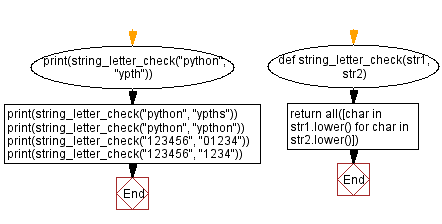﻿ Python: Test if the letters in the second string are present in the first string - w3resource# Python: Test if the letters in the second string are present in the first string

## Python Basic - 1: Exercise-88 with Solution

Write a Python program that accept two strings and test if the letters in the second string are present in the first string.

Sample Solution:

Python Code:

``````def string_letter_check(str1, str2):
return all([char in str1.lower() for char in str2.lower()])
print(string_letter_check("python", "ypth"))
print(string_letter_check("python", "ypths"))
print(string_letter_check("python", "ypthon"))
print(string_letter_check("123456", "01234"))
print(string_letter_check("123456", "1234"))
``````

Sample Output:

```True
False
True
False
True
```

Pictorial Presentation:Flowchart:Python Code Editor:

Have another way to solve this solution? Contribute your code (and comments) through Disqus.

What is the difficulty level of this exercise?

Test your Programming skills with w3resource's quiz.

﻿

## Python: Tips of the Day

What is the difference between Python's list methods append and extend?

append: Appends object at the end.

```x = [1, 2, 3]
x.append([4, 5])
print (x)
```

Output:

```[1, 2, 3, [4, 5]]
```

extend: Extends list by appending elements from the iterable.

```x = [1, 2, 3]
x.extend([4, 5])
print (x)
```

Output:

```[1, 2, 3, 4, 5]
```

Ref: https://bit.ly/2AZ6ZFq